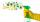# Two fraction equation

Find d value of q in the equation 30/16=q/48.

q =  90

### Step-by-step explanation:Did you find an error or inaccuracy? Feel free to write us. Thank you!Tips to related online calculators
Need help to calculate sum, simplify or multiply fractions? Try our fraction calculator.
Do you have a linear equation or system of equations and looking for its solution? Or do you have a quadratic equation?

## Related math problems and questions:

• Over fractionSolve equation: p over 5 equals fraction numerator 7 p over denominator 6 end fraction plus 5
• TimeWrite time in minutes rounded to one decimal place: 3 h 48 m 45 s.
• Equation with fractionsWhat is the simplified form of the equation fraction 4 over 5 n minus fraction 1 over 5 equals fraction 2 over 5 n? n = -2 n = 4 n = fraction 1 over 2
• The rawThe raw data presented here are the scores (out of 100 marks) of a market survey regarding the acceptability of new product launched by a company for random sample of 50 respondents: 40 45 41 45 45 30 30 8 48 25 26 9 23 24 26 29 8 40 41 42 39 35 18 25 35
• Speed of Slovakian trainsRudolf decided to take the train from the station 'Ostratice' to 'Horné Ozorovce'. In the train timetables found train Os 5409 : km 0 Chynorany 15:17 5 Ostratice 15:23 15:23 8 Rybany 15:27 15:27 10 Dolné Naštice 15:31 15:31 14 Bánovce nad Bebravou 15:35 1
• A class IV.CIn a class 2/5 were boys. 30 were girls. How many more girls than boys are there?
• A fractionI think of a fraction, if we increase its numerator and denominator by one, the value of the fraction increases by one tenth, what fraction do I think?
• Two numbers 11The sum of two rational numbers is (-2). If one of them is 3/5, find the other.
• FractionFind for what x fraction (-4x -6)/(x) equals:
• Fractions and mixed numerals(a) Convert the following mixed numbers to improper fractions. i. 3 5/8 ii. 7 7/6 (b) Convert the following improper fraction to mixed number. i. 13/4 ii. 78/5 (c) Simplify these fractions to their lowest terms. i. 36/42 ii. 27/45 2. evaluate following ex
• CompareCompare fractions (34)/(3) and (12)/(4). Which fraction of the lower?
• If-then equationIf 1/2 + 2/5s = s - 3/4 what is the value of s?
• Simplest form of a fractionWhich one of the following fraction after reducing in simplest form is not equal to 3/2? a) 15/20 b) 12/8 c) 27/18 d) 6/4
• Guess a fractionTom was asked to guess a fraction. The sum of 1/2 the numerator and 1/3 of its denominator is 30. If Tom subtracts 36 from its denominator, the fraction becomes 1/3. What is the fraction that Tom was asked to guess? (Leave your answer in simplest form)
• Find the 11Find the quotient of 229.12 and 12.32
• Two roomsWoman cleans the room in 30 minutes a man in 90 minutes. How long will take they clean two rooms?
• Percent calculationCalculate 8% if 44% is 32.